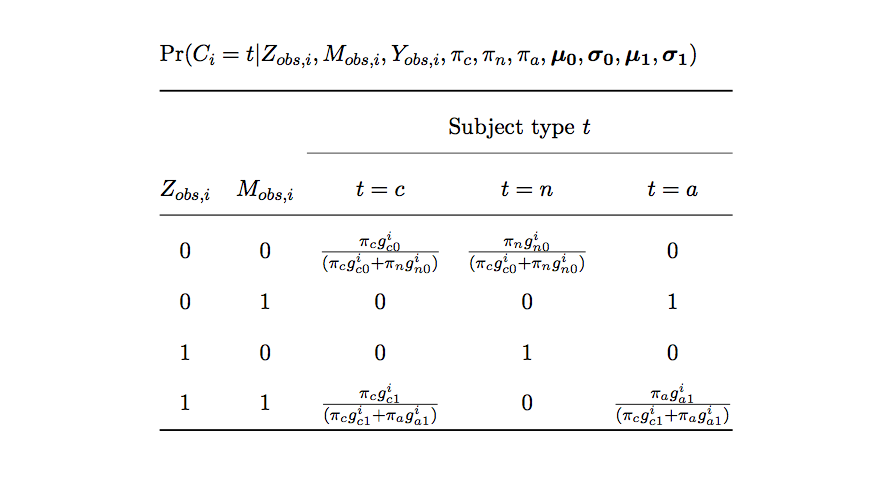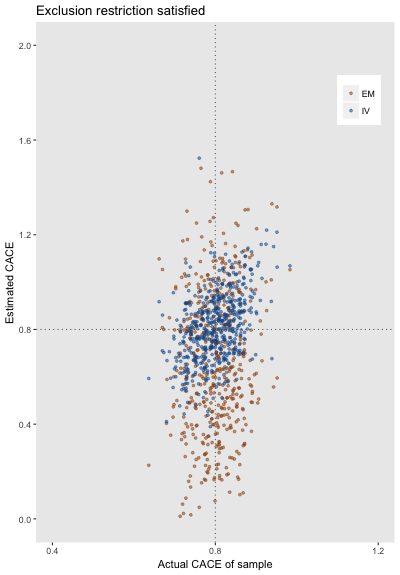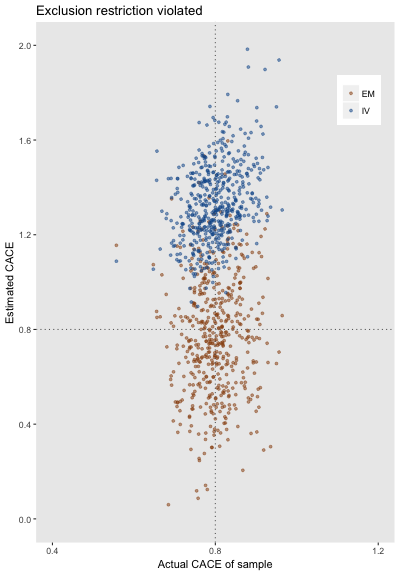# CACE closed: EM opens up exclusion restriction (among other things)

This is the third, and probably last, of a series of posts touching on the estimation of complier average causal effects (CACE) and latent variable modeling techniques using an expectation-maximization (EM) algorithm. What follows is a simplistic way to implement an EM algorithm in R to do principal strata estimation of CACE.

### The EM algorithm

In this approach, we assume that individuals fall into one of three possible groups - never-takers, always-takers, and compliers - but we cannot see who is who (except in a couple of cases). For each group, we are interested in estimating the unobserved potential outcomes $$Y_0$$ and $$Y_1$$ using observed outcome measures of $$Y$$. The EM algorithm does this in two steps. The E-step estimates the missing class membership for each individual, and the M-step provides maximum likelihood estimates of the group-specific potential outcomes and variation.

An estimate group membership was presented in this Imbens & Rubin 1997 paper. The probability that an individual is a member of a particular group is a function of how close the individual’s observed outcome is to the mean of the group and the overall probability of group membership:where $$Z$$ is treatment assignment and $$M$$ is treatment received. In addition, $$g_{c0}^i = \phi\left( \frac{Y_{obs,i} - \mu_{c0}}{\sigma_{c0}} \right)/\sigma_{c0}$$, where $$\phi(.)$$ is the standard normal density. (And the same goes for the other $$g^i$$’s.) $$\pi_a$$, $$\pi_n$$, and $$\pi_c$$ are estimated in the prior stage (or with starting values). $$\mu_{c0}$$, $$\mu_{c1}$$, $$\sigma_{c0}$$, $$\sigma_{c1}$$, etc. are also estimated in the prior M-step or with starting values in the case of the first E-step. Note that because we are assuming monotonicity (no deniers - which is not a necessary assumption for the EM approach, but used here to simplify things a bit), the probability of group membership is 1 for those randomized to control but who receive treatment (always-takers) and for those randomized to intervention but refuse (never-takers).

### EM steps

I’ve created a separate function for each step in the algorithm. The E-step follows the Imbens & Rubin specification just described. The M-step just calculates the weighted averages and variances of the outcomes within each $$Z$$/$$M$$ pair, with the weights coming from the probabilities estimated in the E-step. (These are, in fact, maximum likelihood estimates of the means and variances.) There are a pair of functions to estimate the log likelihood after each iteration. We stop iterating once the log likelihood has reached a stable state. And finally, there is a function to initialize the 15 parameters.

One thing to highlight here is that a strong motivation for using the EM algorithm is that we do not need to assume the exclusion restriction. That is, it is possible that randomizing someone to the intervention may have an effect on the outcome even if there is no effect on whether or not the intervention is used. Or in other words, we are saying it is possible that randomization has an effect on always-takers and never-takers, an assumption we cannot make using an instrumental variable (IV) approach. I mention that here, because the M-step function as written here explicitly drops the exclusion restriction assumption. However, I will first illustrate the model estimates in a case where data are indeed based on that assumption; while my point is to show that the EM estimates are unbiased as are the IV estimates in this scenario, I may actually be introducing a small amount of bias into the EM estimate by not re-writing the function to create a single mean for always-takers and never-takers. But, for brevity’s sake, this seems adequate.

estep <- function(params, y, z, m) {

piC <- 0
piN <- 0
piA <- 0

if (z == 0 & m == 0) {

gC0 <- dnorm((y - params$mC0)/params$sC0) / params$sC0 gN0 <- dnorm((y - params$mN0)/params$sN0) / params$sN0

piC <- params$pC * gC0 / ( params$pC * gC0 + params$pN * gN0) piN <- 1- piC } if (z == 0 & m == 1) { piA <- 1 } if (z == 1 & m == 0) { piN <- 1 } if (z == 1 & m == 1) { gC1 <- dnorm((y - params$mC1)/params$sC1) / params$sC1
gA1 <- dnorm((y - params$mA1)/params$sA1) / params$sA1 piC <- params$pC * gC1 / ( params$pC * gC1 + params$pA * gA1)
piA <- 1 - piC
}

return(list(piC = piC, piN = piN, piA = piA))

}

library(Weighted.Desc.Stat)

mstep <- function(params, dx) {

params$mN0 <- dx[z == 0 & m == 0, w.mean(y, piN)] # never-taker params$sN0 <- dx[z == 0 & m == 0, sqrt(w.var(y, piN))] # never-taker

params$mN1 <- dx[z == 1 & m == 0, w.mean(y, piN)] # never-taker params$sN1 <- dx[z == 1 & m == 0, sqrt(w.var(y, piN))] # never-taker

params$mA0 <- dx[z == 0 & m == 1, w.mean(y, piA)]# always-taker params$sA0 <- dx[z == 0 & m == 1, sqrt(w.var(y, piA))] # always-taker

params$mA1 <- dx[z == 1 & m == 1, w.mean(y, piA)]# always-taker params$sA1 <- dx[z == 1 & m == 1, sqrt(w.var(y, piA))] # always-taker

params$mC0 <- dx[z == 0 & m == 0, w.mean(y, piC)] # complier, z=0 params$sC0 <- dx[z == 0 & m == 0, sqrt(w.var(y, piC))] # complier, z=0

params$mC1 <- dx[z == 1 & m == 1, w.mean(y, piC)] # complier, z=1 params$sC1 <- dx[z == 1 & m == 1, sqrt(w.var(y, piC))] # complier, z=1

nC <- dx[, sum(piC)]
nN <- dx[, sum(piN)]
nA <- dx[, sum(piA)]

params$pC <- (nC / sum(nC, nN, nA)) params$pN <- (nN / sum(nC, nN, nA))
params$pA <- (nA / sum(nC, nN, nA)) return(params) } like.i <- function(params, y, z, m) { if (z == 0 & m == 0) { l <- params$pC * dnorm(x = y, mean = params$mC0, sd = params$sC0) +
params$pN * dnorm(x = y, mean = params$mN0, sd = params$sN0) } if (z == 0 & m == 1) { l <- params$pA * dnorm(x = y, mean = params$mA0, sd = params$sA0)
}

if (z == 1 & m == 0) {
l <- params$pN * dnorm(x = y, mean = params$mN1, sd = params$sN1) } if (z == 1 & m == 1) { l <- params$pC * dnorm(x = y, mean = params$mC1, sd = params$sC1) +
params$pA * dnorm(x = y, mean = params$mA1, sd = params$sA1) } return(l) } loglike <- function(dt, params){ dl <- dt[, .(l.i = like.i(params, y, z, m)), keyby = id] return(dl[, sum(log(l.i))]) } initparams <- function() { params = list(pC = 1/3, pN = 1/3, pA = 1/3, mC0 = rnorm(1,0,.1), sC0 = 0.2, mC1 = rnorm(1,0,.1), sC1 = 0.2, mN0 = rnorm(1,0,.1), sN0 = 0.2, mN1 = rnorm(1,0,.1), sN1 = 0.2, mA0 = rnorm(1,0,.1), sA0 = 0.2, mA1 = rnorm(1,0,.1), sA1 = 0.2) return(params) } ### Data defintions These next set of statements define the data that will be generated. I define the distribution of group assignment as well as potential outcomes for the intervention and the outcome $$Y$$. We also define how the observed data will be generated, which is a function of treatment randomization … library(simstudy) ### Define data distributions # Status : # 1 = A(lways taker) # 2 = N(ever taker) # 3 = C(omplier) def <- defDataAdd(varname = "Status", formula = "0.25; 0.40; 0.35", dist = "categorical") # potential outcomes (PO) for intervention depends on group status def <- defDataAdd(def, varname = "M0", formula = "(Status == 1) * 1", dist = "nonrandom") def <- defDataAdd(def, varname = "M1", formula = "(Status != 2) * 1", dist = "nonrandom") # observed intervention status based on randomization and PO def <- defDataAdd(def, varname = "m", formula = "(z==0) * M0 + (z==1) * M1", dist = "nonrandom") # potential outcome for Y (depends group status - A, N, or C) # under assumption of exclusion restriction defY0 <- defCondition(condition = "Status == 1", formula = 0.3, variance = .25, dist = "normal") defY0 <- defCondition(defY0, condition = "Status == 2", formula = 0.0, variance = .36, dist = "normal") defY0 <- defCondition(defY0, condition = "Status == 3", formula = 0.1, variance = .16, dist = "normal") defY1 <- defCondition(condition = "Status == 1", formula = 0.3, variance = .25, dist = "normal") defY1 <- defCondition(defY1, condition = "Status == 2", formula = 0.0, variance = .36, dist = "normal") defY1 <- defCondition(defY1, condition = "Status == 3", formula = 0.9, variance = .49, dist = "normal") # observed outcome function of actual treatment defy <- defDataAdd(varname = "y", formula = "(z == 0) * Y0 + (z == 1) * Y1", dist = "nonrandom") ### Data generation I am generating multiple data sets and estimating the causal effects for each using the EM and IV approaches. This gives better picture of the bias and variation under the two different scenarios (exclusion restriction & no exclusion restriction) and different methods (EM & IV). To simplify the code a bit, I’ve written a function to consolidate the data generating process: createDT <- function(n, def, defY0, defY1, defy) { dt <- genData(n) dt <- trtAssign(dt, n=2, grpName = "z") dt <- addColumns(def, dt) genFactor(dt, "Status", labels = c("Always-taker","Never-taker", "Complier"), prefix = "A") dt <- addCondition(defY0, dt, "Y0") dt <- addCondition(defY1, dt, "Y1") dt <- addColumns(defy, dt) } set.seed(16) dt <- createDT(2500, def, defY0, defY1, defy) options(digits = 3) dt ## id Y1 Y0 z Status M0 M1 m AStatus y ## 1: 1 0.8561 -0.2515 0 3 0 1 0 Complier -0.2515 ## 2: 2 -0.3264 0.2694 0 2 0 0 0 Never-taker 0.2694 ## 3: 3 0.0757 -0.4576 1 2 0 0 0 Never-taker 0.0757 ## 4: 4 -0.3660 0.3868 0 2 0 0 0 Never-taker 0.3868 ## 5: 5 0.6103 0.6735 0 3 0 1 0 Complier 0.6735 ## --- ## 2496: 2496 2.8298 0.6357 1 3 0 1 1 Complier 2.8298 ## 2497: 2497 0.1712 1.1899 0 1 1 1 1 Always-taker 1.1899 ## 2498: 2498 0.3844 -0.4837 1 2 0 0 0 Never-taker 0.3844 ## 2499: 2499 1.8602 0.3124 1 3 0 1 1 Complier 1.8602 ## 2500: 2500 -0.2857 0.0227 0 1 1 1 1 Always-taker 0.0227 ### CACE estimation Finally, we are ready to put all of this together and estimate the CACE using the EM algorithm. After initializing the parameters (here we just use random values except for the probabilities of group membership, which we assume to be 1/3 to start), we loop through the E and M steps, checking the change in log likelihood each time. For this single data set, we provide a point estimate of the CACE using EM and IV. (We could provide an estimate of standard error using a bootstrap approach.) We see that both do a reasonable job, getting fairly close to the truth. params <- initparams() prev.loglike <- -Inf continue <- TRUE while (continue) { dtPIs <- dt[, estep(params, y, z, m), keyby = id] dx <- dt[dtPIs] params <- mstep(params, dx) EM.CACE <- params$mC1 - params\$mC0

current.loglike <- loglike(dt, params)
diff <- current.loglike - prev.loglike
prev.loglike <- current.loglike
if ( diff < 1.00e-07 ) continue = FALSE

}

library(ivpack)
ivmodel <- ivreg(formula = y ~ m | z, data = dt, x = TRUE)

data.table(truthC = dt[AStatus == "Complier", mean(Y1 - Y0)],
IV.CACE = coef(ivmodel),
EM.CACE)
##    truthC IV.CACE EM.CACE
## 1:  0.808   0.825   0.309

### More general performance

I am not providing the code here (it is just a slight modification of what has come before), but I want to show the results of generating 1000 data sets of 500 observations in each. The first plot assumes all data sets were generated using an exclusion restriction - just as we did with the single data set. The IV approach, as expected is unbiased (estimated bias 0.01), while the EM approach is slightly biased (-0.13). We can also see that the EM approach (standard deviation 0.30) has more variation than IV (standard deviation 0.15), while the actual sample CACE (calculated based on the actual group membership and potential outcomes) had a standard deviation of 0.05, which we can see from the narrow vertical band in the plot:In the second set of simulations, I change the potential outcomes definition so that the exclusion restriction is no longer relevant.

defY0 <- defCondition(condition = "Status == 1",
formula = 0.3, variance = .20, dist = "normal")
defY0 <- defCondition(defY0, condition = "Status == 2",
formula = 0.0, variance = .36, dist = "normal")
defY0 <- defCondition(defY0, condition = "Status == 3",
formula = 0.1, variance = .16, dist = "normal")

defY1 <- defCondition(condition = "Status == 1",
formula = 0.7, variance = .25, dist = "normal")
defY1 <- defCondition(defY1, condition = "Status == 2",
formula = 0.2, variance = .40, dist = "normal")
defY1 <- defCondition(defY1, condition = "Status == 3",
formula = 0.9, variance = .49, dist = "normal")

In this second case, the IV estimate is biased (0.53), while the EM estimated does quite well (-.03). (I suspect EM did worse in the first example above, because estimates were made without the assumption of the exclusion restriction, even though that was the case.) However, EM estimates still have more variation than IV: standard deviation 0.26 vs 0.17, consistent with the estimates under the exclusion restriction assumption. This variation arises from the fact that we don’t know what the true group membership is, and we need to estimate it. Here is what the estimates look like:### Can we expand on this?

The whole point of this was to illustrate that there might be a way around some rather restrictive assumptions, which in some cases might not seem so reasonable. EM methods provide an alternative way to approach things - more of which you can see in the free online course that inspired these last few posts. Unfortunately, there is no obvious way to tackle these problems in R using existing packages, and I am not suggesting that what I have done here is the best way to go about it. The course suggests using Mplus. While that is certainly a great software package, maybe it would be worthwhile to build an R package to implement these methods more completely in R? Or maybe someone has already done this, and I just haven’t come across it yet?